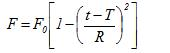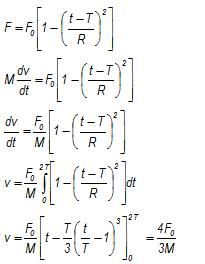Courses

# Test: Newton’s First Law Of Motion

## 10 Questions MCQ Test Physics For JEE | Test: Newton’s First Law Of Motion

Description
This mock test of Test: Newton’s First Law Of Motion for JEE helps you for every JEE entrance exam. This contains 10 Multiple Choice Questions for JEE Test: Newton’s First Law Of Motion (mcq) to study with solutions a complete question bank. The solved questions answers in this Test: Newton’s First Law Of Motion quiz give you a good mix of easy questions and tough questions. JEE students definitely take this Test: Newton’s First Law Of Motion exercise for a better result in the exam. You can find other Test: Newton’s First Law Of Motion extra questions, long questions & short questions for JEE on EduRev as well by searching above.
QUESTION: 1

### If the net external force on the body is zero, its

Solution:

As F = ma,

and  F= 0,

therefore, a = 0 as m cannot be 0.

QUESTION: 2

### When a bull pulls a cart, the force that helps the bull to move forward is the force exerted by

Solution:

Bull exert the force on the ground , and ground exert equal force on cart and bull as well which help them to move forward

QUESTION: 3

### Dust can be removed out of a blanket using

Solution:

When a blanket is bitten with a stick or something then particles of blanket come into motion while dust particles remains in rest thus comes out which shows law of inertia

QUESTION: 4

Which law governs the phenomenon that a tablecloth is pulled out from under a setting of chinaware without damaging it?

Solution:

According to Newton’s first Law, the cloth is set in motion but the chinaware continues to remain in its state of rest; and hence it is not damaged.

QUESTION: 5

Two masses are in the ratio 1:5. What is ratio of their inertia?

Solution:

Force of inertia = ma

Let the masses be 1x and 5x

Force of inertia for 1st body= 1x * a

Force of inertia for 2nd = 5x * a

Ratio= x * a / 5x * a = 1:5

QUESTION: 6

We slip on a rainy day due to ______.

Solution:

In rainy day, the rain water forms a layer on the road. This layer works as a lubricant which decreases the friction. Due to less friction, we slip on rainy day.

QUESTION: 7

A 6 kg object is subject to three forces
F1 = 20+ 30j N
F2 = 8i - 50j N
F3 = 2i + 2j N
Find the acceleration of object.

Solution:

F = F1 + F2 + F3 = 30i - 18j
F = ma
Substituting F and m = 6 kg (given) in the above equation we get,
a = 5i - 3j
Hence (b) is correct choice

QUESTION: 8

Passengers in a bus lean forward as bus suddenly stops. This is due to

Solution:

This is due to the presence of inertia.

When the bus moves, the passenger's body comes in the state of motion but when the bus stops the lower part of the body which is in contact with floor comes in the state of rest whereas the upper part of body still remains in the state of motion and because of this the upper part of the body falls in the forward direction.

QUESTION: 9

A particle of mass M, originally at rest is subjected to a force whose direction is constant but whose magnitude varies with the time according to the relationwhere F0 and T are constant. The force acts only for the time interval 2T. Find the velocity v of the particle after time 2T.

Solution:Hence (b) is the correct choice.

QUESTION: 10

How is inertia used when riding a bicycle?

Solution:

Even if we stop paddling due to inertia of motion we will continue to move forward  upto a certain distance as friction will finally make us stop.